Related Articles

# Mean and Mode in SQL Server

• Last Updated : 28 Mar, 2018

Mean is the average of the given data set calculated by dividing the total sum by the number of values in data set.

Example:

```Input:  1, 2, 3, 4, 5
Output: 3
Explanation:
sum = 1 + 2 + 3 + 4 + 5 = 15
number of values = 5
mean = 15 / 5 = 3
```

Query to find mean in the table

```SELECT Avg(Column_Name)
FROM  Table_Name
```

Example:

Creating Table: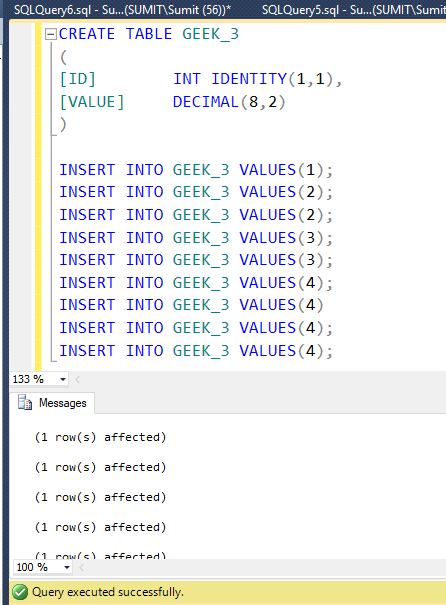Table Content: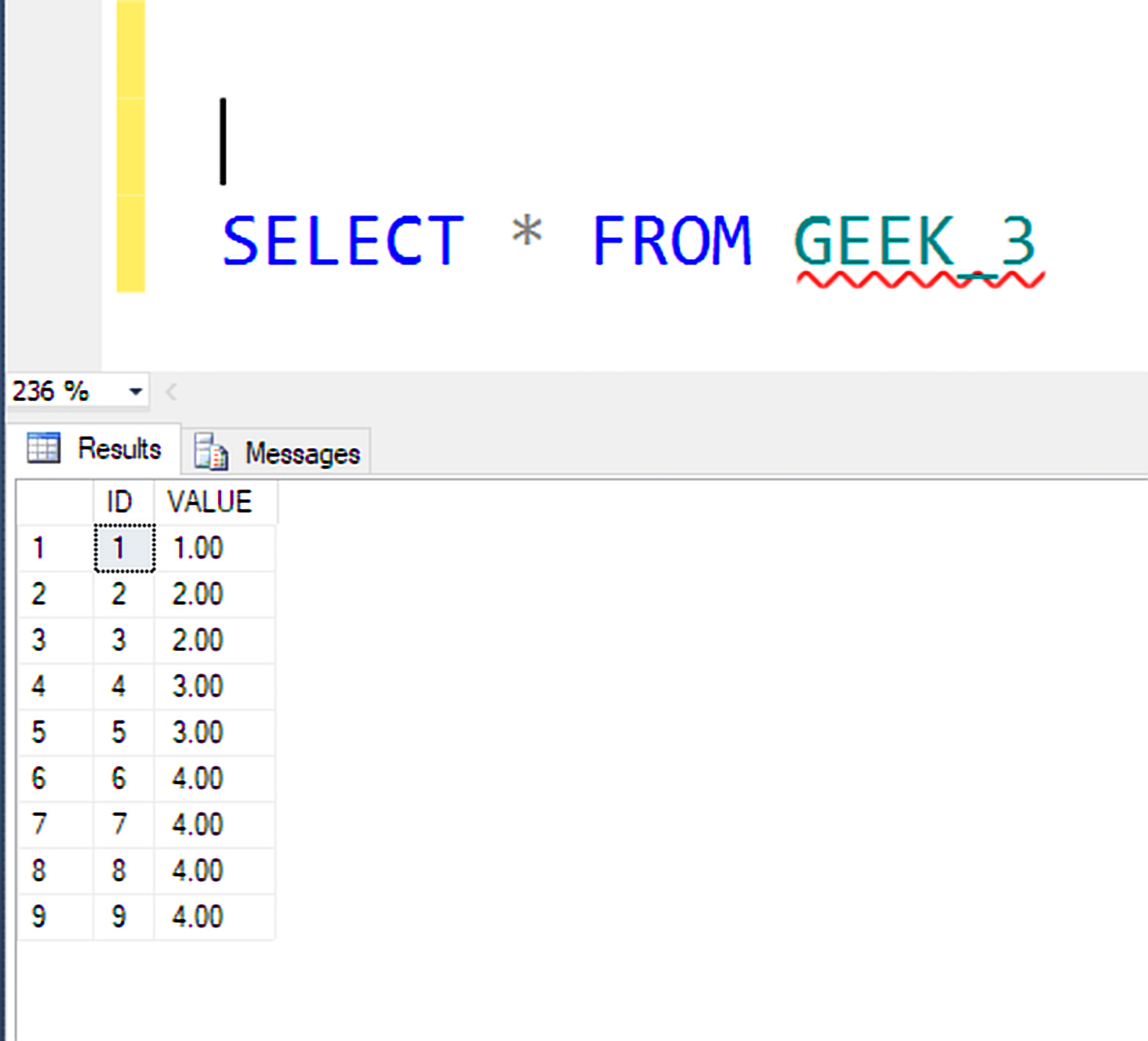Query to find Mean: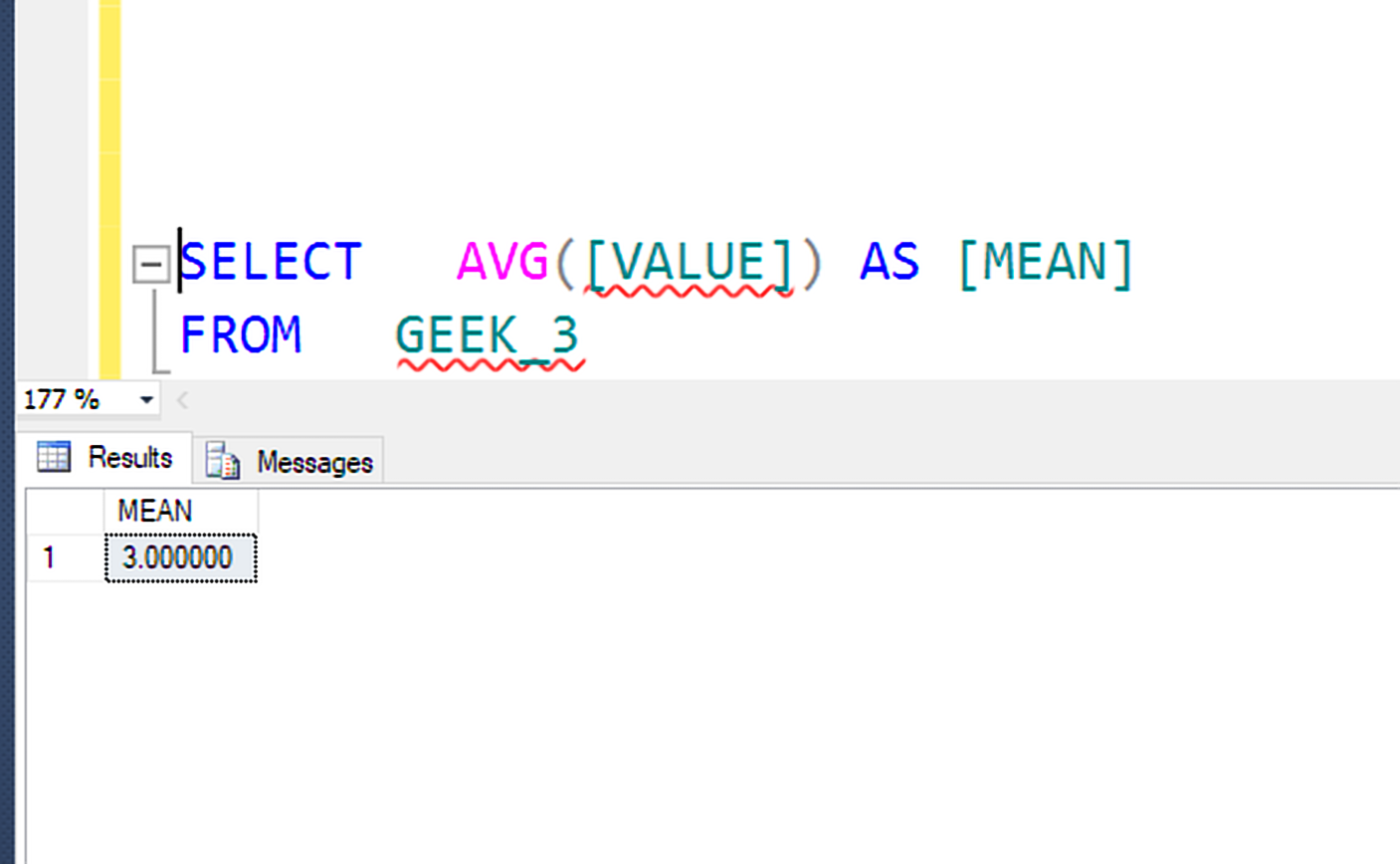Mode of a data set is the value that appears most frequently in a series of data.

Example:

```Input:  1, 2, 2, 3, 4, 4, 4, 5, 5, 6
Output: 4
Explanation:
2 occurs 2 times, 5 occurs 2 times
4 occurs 3 times and rest other
occurs 1 time
So, 4 occurs most frequently and
is thus the output.
```

Query to find mode in the table

```SELECT TOP 1 Column_Name
FROM   Table_name
GROUP  BY [Column_Name]
ORDER  BY COUNT(*) DESC
```

Example:

Creating Table: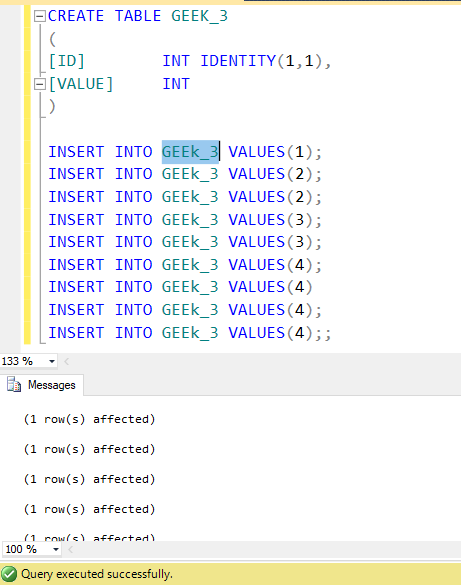Table Content: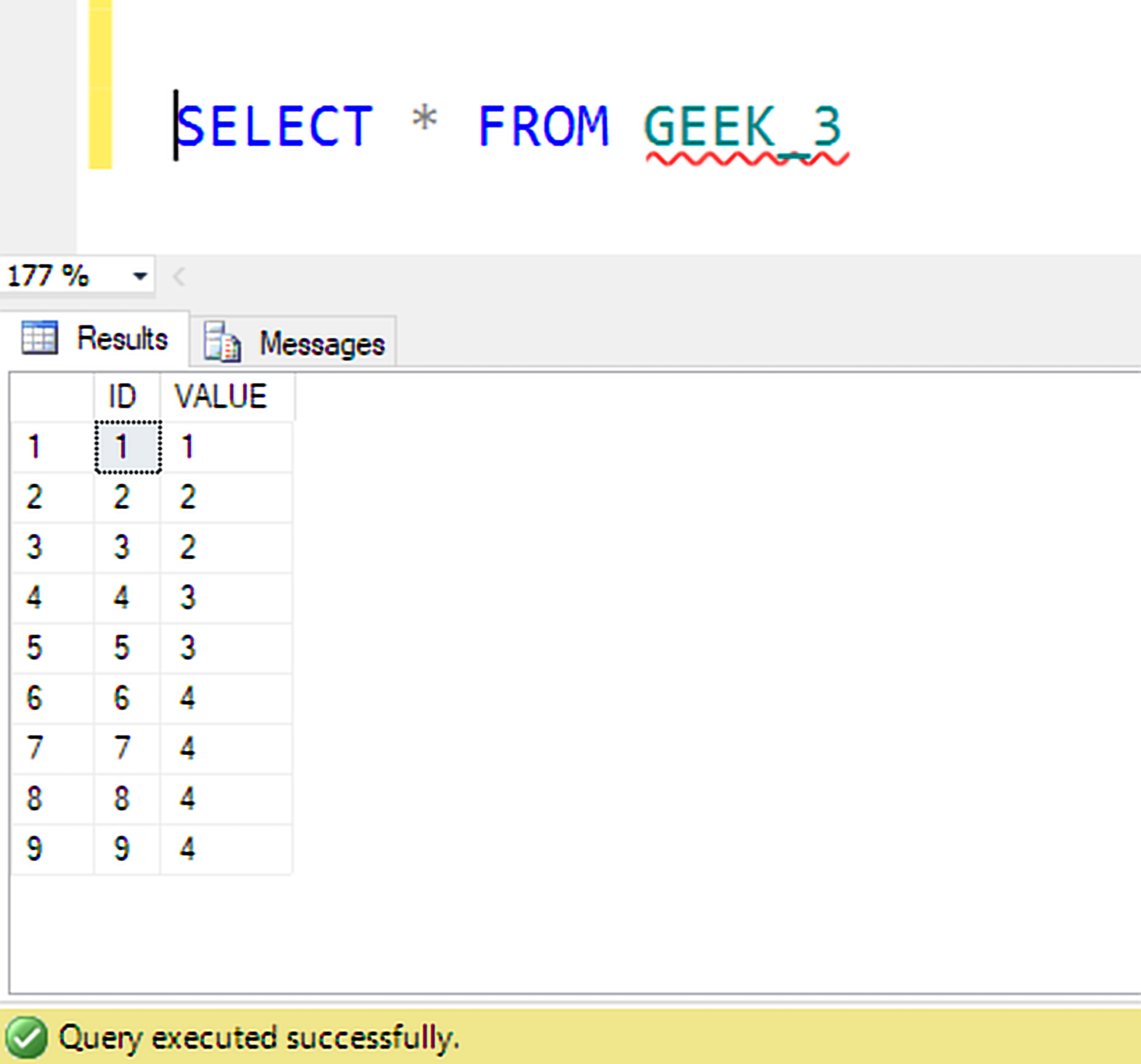Query to find Mode: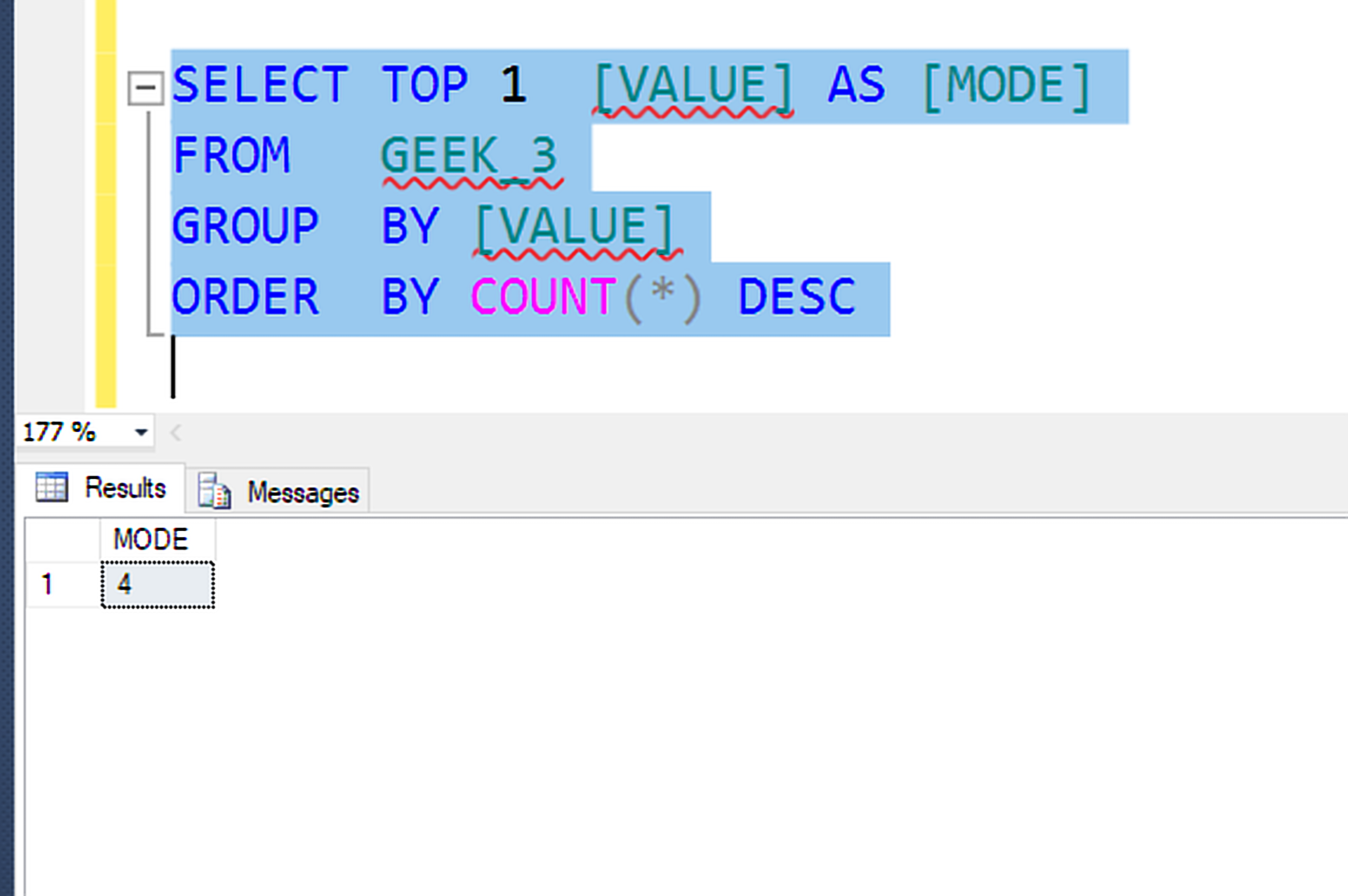Attention reader! Don’t stop learning now. Learn SQL for interviews using SQL Course  by GeeksforGeeks.

My Personal Notes arrow_drop_up